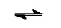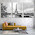Solving Weak Acid and Weak Base pH problems | Chemistry Net

# Solving Weak Acid and Weak Base pH problems

Solving Weak Acid and Weak Base pH problems

# Solving Weak Acid and Weak Base pH problemsFig. I.1: Calculating the pH of a weak acid
In a previous post entitled “Weak Acids and Bases – Calculate the pH of a weak acid” a general equation was derived for the calculation of [H+] in weak acid (base) solutions (mathematical approach).  A four-step method was also proposed for solving weak acid base problems and for the calculation of the pH of a weak acid or base (chemical approach) (Fig. I.1).
Some extra solved examples on weak acid (base) chemistry are shown below. The “chemical approach” method is used for the solution.

#### Example I.1

What the concentration of an acidic solution ΗΑ must be so that
[H+]= 3.5 * 10-4 M (ka =1,7 * 10-5)

STEP
RESULT

 GIVEN [H+]= 3.5 * 10-4 M ka =1.7 * 10-5 UNKNOWN [HΑ] = ;

I

HA is a weak acid as the ka value shows.
Write the ionization equilibrium reaction:

HΑΗ+    +   Α-            (1)

II

Write down the equilibrium constant expression and the equilibrium constant:

ka =  +] . [A-] / [HA] = 1.7 * 10-5   (2)

III

Let us suppose that y M is the initial concentration of HA. Then the equilibrium concentrations of the species involved are as follows:

 Initial y Μ 0 Μ 0 Μ Change -3.5 * 10-4 +3.5 * 10-4 +3.5 * 10-4 Final (at equilibrium) (y – 3.5 * 10-4) 3.5 * 10-4 3.5 * 10-4

IV

From (2) and the equilibrium concentrations from the above table:
ka = [Η+] . [A-] / [HA] = (3.5 * 10-4)2  / (y-3.5 * 10-4)  = 1.7 * 10-5
and y = 7.2 * 10-3 M
Therefore  [HΑ] = 7.2 * 10-3 M

#### Example I.2

A weak acid solution is prepared by dissolving 8.3 * 10-1 g of HA in water. The final volume of the solution is measured to be 100 ml. What is the equilibrium concentration of the species involved? (kΗΑ = 1.8 * 10-4, ΜΒΗΑ = 46 g/mol).

STEP
RESULTS

 DATA mHA = 8.3 * 10-1 g VΔ = 100 ml kHA = 1.8 * 10-4 ΜΒΗΑ = 46 g/mol UNKNOWN [HΑ] = ?  [Η+] = ?  [A-] = ?

I

HA is a weak acid as we can see from kHA. Therefore, it dissociates partially in water.
Write the ionization equilibrium reaction:

HΑΗ+    +   Α-            (1)

Calculate the moles of  ΗΑ in solution:
nHA = 8.3 * 10-1 g /46 g/mol  = 1.8 * 10-2 mol

The initial concentration of ΗΑ is calculated as follows:
100 ml solution  contain  1,8 . 10-2 mol ΗΑ
1000 ml solution contain      y = ; mol ΗΑ
y = [HΑ]initial =1.8 * 10-1 mol/ℓ   (2)

II

Write down the equilibrium constant expression and the equilibrium constant:

kHA =  +] . [A-] / [HA] = 1,8 . 10-4   (3)

III

Let us suppose that x moles ΗΑ dissociate. The equilibrium concentration of the species involved is given below:

 HΑ Η+ Α- Initial 0.18 Μ 0 0 Change -x M +x M +x M Final (at equilibrium) (στην χημ. ισορρ.) (0.18-x) M +x M +x M

IV

From (3) and the equilibrium concentrations of the species involved:
ka =  [Η+] . [A-] / [HA] = (x)2 / (0,18-x ) = 1.8 * 10-4
(x)2 /0.18 = 1.8 * 10-4
x = 5.7 * 10-3 M  (4)  (assuming that 0,18-x ≈ 0,18 since ΗΑ is a weak acid and it partially dissociates in water. Therefore the value of x will be small compared to the initial concentration of ΗΑ)

Checking for the validity of the above approximation: If the value of x is less than 5% of the initial concentration of HA the approximation 0,18-x ≈ 0,18 is valid.

5.7 * 10-3 * 100 / 0.18  = 3.2%

#### Example I.3

The ionization constant of NH3 in water is 10-5. Calculate the following for a 0.1     M NH3 solution: a) the degree of dissociation α  b) the concentration of [OH-] in solution

STEP
RESULTS

 DATA ΝΗ3 kb = 10-5 [ΝΗ3] = 0.1 M UNKNOWNS α = ?  [ΟΗ-] = ?
I
ΝΗ3 is a weak base as the value of kb shows. Therefore, it dissociates partially in water according to:

H2O   +      ΝΗ3ΝΗ4+    +    ΟΗ-   (1)

Let us suppose that α is the degree of dissociation of ΝΗ3 in water. Therefore:
α = moles ΝΗ3 that dissociate / total moles ΝΗ3
Therefore moles ΝΗ3 that dissociate = 0.1 * α    (2)

II
Write down the equilibrium constant expression and the equilibrium constant:

kb =  [NΗ4+] . [OH-] / [NH3] = 10-5   (3)

III

The equilibrium concentration of the species involved is given below:

 ΝΗ3 ΝΗ4+ ΟΗ- Initial 0.1 Μ 0 0 Change (0.1-0.1α)  M +0.1 * α M +0.1 * α M Final (at equilibrium) 0.1 * (1-α) M 0.1 * α M +0.1 * α M

IV
From (3) and the above equilibrium concentrations of the species involved:
Kb = (0.1 * α). (0.1 * α) / 0.1 * (1-α) = 10-5 = 0.01 * α2 / 0.1 = 10-5
Therefore,  α = 0,01 (4)
Assuming that (1-α) ≈ 1 since the value of α is small compared to [ΝΗ3] = 0.1 M

Therefore [OH-] = 0.1 *α = 0.1 * 0.01 = 10-3 M

Therefore  α = 0.01 and [OH-] = 10-3 M

Relevant Posts

References

J-L. Burgot “Ionic Equilibria in Analytical Chemistry”, Springer Science & Business Media, 2012
J.N. Butler  “Ionic Equilibrium – Solubility and pH calculations”, Wiley – Interscience, 1998
Clayden, Greeves, Waren and Wothers “Organic Chemistry”, Oxford,
D. Harvey,  “Modern Analytical Chemistry”, McGraw-Hill Companies Inc., 2000
Toratane Munegumi, World J. of Chem. Education, 1.1, 12-16 (2013)
J.N. Spencer et al., “Chemistry structure and dynamics”, 5th Edition, John Wiley & Sons, Inc., 2012
L. Cardellini, Chem. Educ. Res. Pract. Eur., 1, 151-160, (2000)

#### 1 comment:

1.If the products you look for are not in our catalog we would be pleased to offer our custom synthesis service. Direct Black GB